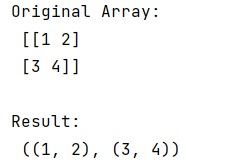# Convert numpy array to tuple

Learn, how to convert numpy array to tuple in Python?
Submitted by Pranit Sharma, on December 24, 2022

NumPy is an abbreviated form of Numerical Python. It is used for different types of scientific operations in python. Numpy is a vast library in python which is used for almost every kind of scientific or mathematical operation. It is itself an array which is a collection of various methods and functions for processing the arrays.

## Converting numpy array to tuple

A tuple is a built-in data type of python used to store heterogeneous elements. A tuple is considered a series or collection and we can perform operations like insert, update, and delete with its elements.

For this purpose, we will first create a NumPy array and then we will map the array into a tuple and convert its type to a tuple.

The map() function is used for applying the given function to each item of a given iterable (list, tuple, etc.) and it returns a map object(which is an iterator).

Let us understand with the help of an example,

## Python code to convert numpy array to tuple

```# Import numpy
import numpy as np

# Creating a numpy array
arr = np.array(((1,2),(3,4)))

# Display original array
print("Original Array:\n",arr,"\n")

# Mapping array into tuple
res = tuple(map(tuple, arr))

# Display result
print("Result:\n",res)
```

Output: